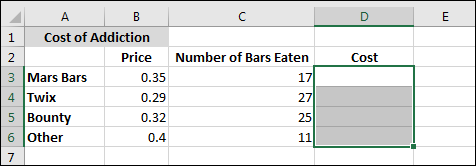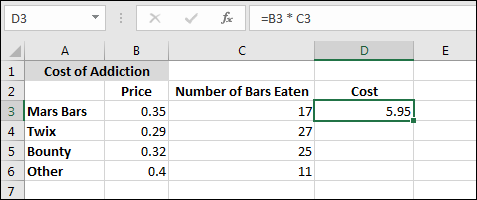# Getting to grips with Array Formulas

An array formula, as its name suggests, is a formula that you use on arrays. Which begs the question, what is an array?

### What is an Array?

An array is a collection of values, rather than a single value. If you had some exam scores in the A column, then all those scores collectively would be called an array of exam scores. Likewise, if you had a column full of car names, then all of those car names together would be an array of car names. So an array is a lot of values treated as one group.

### What is an Array Formula?

An array formula is just a formula that works with arrays (groups of values). As an example, take the Chocolate Addiction spreadsheet you constructed in a previous section. It was like this:So we want to multiply the cost of each chocolate bar by how many we've eaten. The formula to go in the D column in the image above is simple:

=PRICE * NUMBER OF BARS EATEN

The first one (0.35 * 17) gives an answer of 5.95. In cell D3, we could have entered:

= B3 * C3

To get the rest of the Cost column, you could then use AutoFill. This is what you did previously.

However, you can use an Array Formula instead of using Autofill. Try this:

• Create the same spreadsheet as in the image above
• Now highlight the cells D3 to D6:• Click inside the formula bar at the top. Type the following formula: (DON'T press the enter key yet.)

= B3:B6 * C3:C6• With the formula in the formula bar, hold down the SHIFT and CTRL keys on your keyboard. Keep them held down, and then press the enter key. The answers to all four of the Costs should appear in the empty cells:Notice the formula bar now: the formula you entered is now surrounded by curly brackets:

{= B3:B6 * C3:C6}

And that's how you can tell that the cell contains an array formula - it's surrounded by curly brackets. Note that you can't enter the curly brackets yourself. So you couldn't type the formula then type the curly brackets on the left and right. You have to press SHIFT + CTRL then press the enter key to get an array formula.

So how does it work? Well, we used two arrays: one array that grouped all the Prices, and another array that grouped all the Number of bars eaten. The Prices array was in the cells B3 to B6, while the Number array was in cells C3 to C6. We told Excel to use these two arrays in a formula. Specifically, we told Excel to multiply the two arrays together and place the answers in the cells D3 to D6 (the cells we highlighted).

Let's try another example of an array formula. This time, we'll enter an array formula in only one cell.

Highlight the cells D3 to D6. Now press the delete key on your keyboard to get rid of all those array formulas.

Click inside cell D3 and enter the following formula:

= B3 * C3Now use AutoFill to get the answers in cells D4, D5, and D6.

OK, this is the standard way you've been doing things. To get the total, you could click into cell D7 and use the following SUM function:

=SUM(D3:D6)

This would then get you an answer of 26.18 in cell D7. Add some text as a label in cell C7, so that your spreadsheet looks something like this:Now let's use an array formula instead.

Add some text as a label to cell C8: Cost using Array Formula. Now click inside cell D8. Enter the following formula, but don't press the enter key just yet:

=SUM(B3:B6 * C3:C6)

Hold down the SHIFT and CTRL keys. Keep them held down and press the enter key on your keyboard. You should see the same answer, 26.18, appear in cell D8. The formula bar at the top will show the curly brackets surrounding the formula:Notice that the array formula you created doesn't need to use the figures in the D column at all. In fact, if you deleted the numbers in cells D3 to D6 only the Cost using Autofill figure would disappear from cell D7. The Cost using Array Formula would still be there in cell D8. That's because you used two arrays to get the total, the array in cells B3 to B6 and the array in cells C3 to C6.

So if you want to save yourself some typing and Autofilling then think about using an array formula instead, especially if you have groups of numbers to work with.

In the next lesson, you'll see how to use an array formula with something called Frequency Distribution.

<--Back to the Excel Contents Page